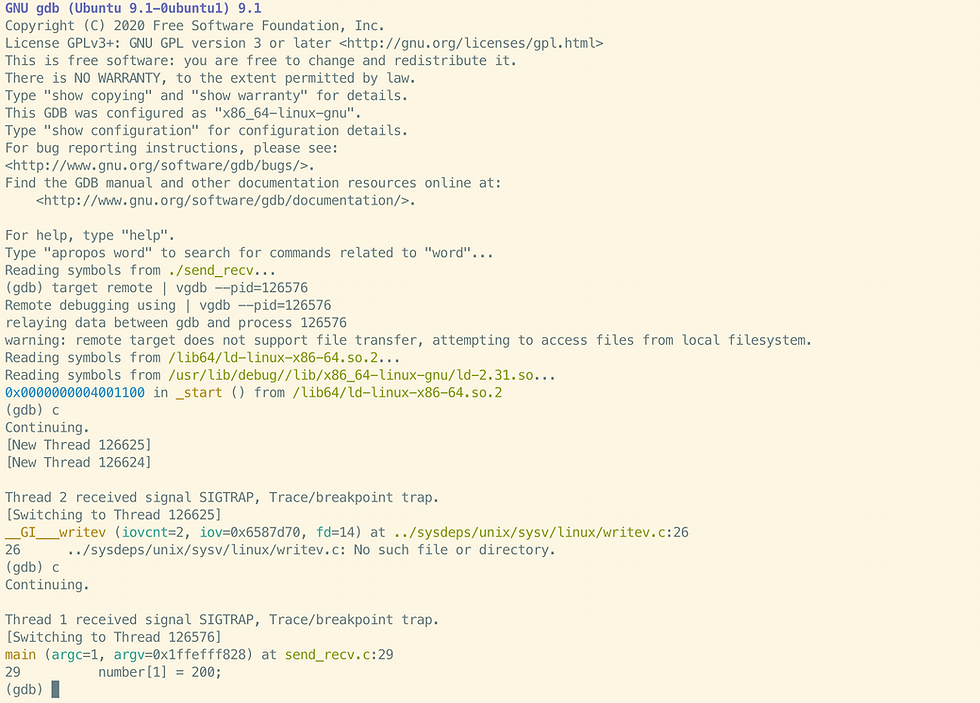top of page
Search
• Joseph

# Debugging MPI Programs Using Valgrind and GDB

Updated: Apr 28, 2022

Debugging a Parallel program is not straightforward as debugging a sequential program because it involves multiple processes with inter-process communication. In this blog post I will be using a simple MPI program with two MPI processes to demonstrate how to use Valgrind and GNU Debugger (GDB) for parallel debugging.

The program is compiled using:

`mpicc send_recv.c -o send_recv`

and it is run using:

`mpirun -np 2 ./send_recv`

When the program is run it generates a segmentation fault:

```
[dolphin:122990] *** Process received signal ***
[dolphin:122990] Signal: Segmentation fault (11)
[dolphin:122990] Signal code: Address not mapped (1)
[dolphin:122990] [ 0] /lib/x86_64-linux-gnu/libc.so.6(+0x46210)
[dolphin:122990] [ 1] ./a.out(+0x1412)[0x55873478b412]
[dolphin:122990] [ 2] /lib/x86_64-linux-gnu/libc.so.6(__libc_start_main+0xf3)
[dolphin:122990] [ 3] ./a.out(+0x120e)[0x55873478b20e]
--------------------------------------------------------------------------Primary job  terminated normally, but 1 process returned a non-zero exit code. Per user-direction, the job has been aborted. ----------------------------------------------------------------------------------------------------------------------------------------------------mpirun noticed that process rank 1 with PID 0 on node dolphin exited on signal 11 (Segmentation fault).--------------------------------------------------------------------------```

First recompile the program using the debug flag and O0 compiler optimisation.

`mpicc -g -O0 send_recv.c -o send_recv`

To debug the program we will have to use three terminals. The first terminal will run Valgind and the other two terminals will run GDB. On the first terminal run the program by attaching Valgrind to the MPI process:

`mpirun -np 2 valgrind --vgdb=yes --vgdb-error=0 ./a.out`

Valgrind will generate the following commands

```==123002== Memcheck, a memory error detector
==123002== Copyright (C) 2002-2017, and GNU GPL'd, by Julian Seward et al.
==123002== Using Valgrind-3.15.0 and LibVEX; rerun with -h for copyright info
==123002== Command: ./a.out
==123002==
==123003== Memcheck, a memory error detector
==123003== Copyright (C) 2002-2017, and GNU GPL'd, by Julian Seward et al.
==123003== Using Valgrind-3.15.0 and LibVEX; rerun with -h for copyright info
==123003== Command: ./send_recv
==123003==
==123002== (action at startup) vgdb me ...
==123002==
==123002== TO DEBUG THIS PROCESS USING GDB: start GDB like this
==123002==   /path/to/gdb ./send_recv
==123002== and then give GDB the following command
==123002==   target remote | /usr/lib/x86_64-linux-gnu/valgrind/../../bin/vgdb --pid=126576
==123002== --pid is optional if only one valgrind process is running
==123002==
==123003== (action at startup) vgdb me ...
==123003==
==123003== TO DEBUG THIS PROCESS USING GDB: start GDB like this
==123003==   /path/to/gdb ./a.out
==123003== and then give GDB the following command
==123003==   target remote | /usr/lib/x86_64-linux-gnu/valgrind/../../bin/vgdb --pid=126577
==123003== --pid is optional if only one valgrind process is running
==123003==```

In this case the two MPI processes are generated with the process id 126577 and 126576 and Valgrind gives clear instructions as how to debug the the individual MPI processes.

```==123002== TO DEBUG THIS PROCESS USING GDB: start GDB like this
==123002==   /path/to/gdb ./send_recv
==123002== and then give GDB the following command
==123002==   target remote | /usr/lib/x86_64-linux-gnu/valgrind/../../bin/vgdb --pid=126576```

So by following the instruction, on the second terminals attach GDB to the executable by running

`gdb ./send_recv`

Once GDB has been launched attach VGDB to the process, inside the GDB, by running the command:

` target remote | vgdb --pid=126576`

Repeat the same in the third terminal but make sure you use the second PID (126577) when you attach VGDB.

Typing c or continue on GDB, on terminal 2 and 3 to progress the MPI Process on both terminals.

MPI Process 1 (third terminal):MPI Process 0 (second terminal):From the GDB, on the second terminal, we can see that there is an error on MPI Process 0. The error occurs in the 29th line in the source code where a data is written to the array. Now if we check the output of the Valgrind on the first terminal we can see that the error is generated because of an invalid write.

Valgrind (first terminal):Now If you inspect the code (you can inspect in from GDB or use the editor) you can see that only one integer space is allocated to the dynamically allocated array but we are trying to write two values to it. In conclusion, the combination of Valgrind and GDB can be used to debug MPI programs if the number of MPI process is small.

###### Problem When Nodes are Allocated Using PBS or SLURM

While the above work with a local machine, you may face problem when you are alloacting nodes using job schedulers like PBS or SLURM. For example here I have allocated a single node using PBS and I am attaching valgrind to that single MPI process (PID of the process is 2294744)Now when I login from another shell to this allocated node and try attaching VGDB to this process I get an error.This is because OpenMPI, which I use here, imposes its own TMPDIR on the processes. So that VGDB coordination FIFOs are located in this TMPDIR directory instead of the /tmp, which is the default TMPDIR for non OpenMPI processes (such as your debugger and VGDB). To resolve this issue I first find the to find the the TMPDIR, before I run my original MPI program. To do this is run

`mpirun -np 1 printenv | grep TMPDIR `

which returns

```TMPDIR=/jobfs/39216350.gadi-pbs

What this means is that the new TMPDIR is /jobfs/39216350.gadi-pbs. Now I can run my original MPI program to attach valgrind to the MPI process.

After this in the second terminal I first run

`export TMPDIR=/jobfs/39216350.gadi-pbs`

Now, if i run GDB and try to attach VGDB everything works.

1. VALGRIND AND GDB: TAME THE WILD

2. How to Debug C Program using gdb in 6 Simple Steps